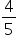Mathematics
Easy

Question

# In the given fraction , 4 is the _____________.

## The correct answer is: Numerator

### Given fractionThe part of a fraction that is above line is called numerator.so, 4 is numerator

Therefore in the given fraction, 4 is the numerator.

### Related Questions to study#### With Turito Foundation.#### Get an Expert Advice From Turito.